Estimating Multiple Derivatives Simultaneously: What Is Optimal? | OMICS International
Journal of Biometrics & Biostatistics

Like us on:

# Estimating Multiple Derivatives Simultaneously: What Is Optimal?

Richard Charnigo* and Cidambi Srinivasan

Department of Statistics, University of Kentucky, Lexington KY 40506-0027

*Corresponding Author:
Richard Charnigo
Department of Statistics
University of Kentucky
Lexington KY 40506-0027
E-mail: [email protected]

Received Date: March 28, 2011; Accepted Date: March 30, 2011; Published Date: March 31, 2011

Citation: Charnigo R, Srinivasan C (2011) Estimating multiple derivatives simultaneously: What is optimal? J Biomet Biostat 2:102e. doi:10.4172/2155-6180.1000102e

Copyright: © 2011 Charnigo R. This is an open-access article distributed under the terms of the Creative Commons Attribution License, which permits unrestricted use, distribution, and reproduction in any medium, provided the original author and source are credited.

Visit for more related articles at Journal of Biometrics & Biostatistics

#### Abstract

Nonparametric regression techniques including kernel smoothing , spline smoothing , and local regression  are useful for estimating a mean response function Âµ(x) in the statistical model Yi = Âµ (xi)+?i when one is unwilling to assume that Âµ(x) is linear (or polynomial of higher but known degree) in the covariate x. These same techniques can also be employed to estimate one or more derivatives of Âµ(x). While the techniques differ in their details, they have a common underlying theme. One specifies a covariate value x0 and estimates Âµ(x) or one of its derivatives at x0 by solving an optimization problem that is localized to a neighborhood of x0, in that only observations with covariate values inside the neighborhood contribute substantively to the solution. For example, the simplest incarnation of this theme is to define Âµ(x0) to be the average of all responses Yi for which |xi-x0| is sufficiently small. As one slides x0 through a continuum of all possible covariate values, an estimated mean response or derivative is then traced out. Selecting the neighborhood size is a crucial implementation decision to which much literature has been devoted.

Nonparametric regression techniques including kernel smoothing , spline smoothing , and local regression  are useful for estimating a mean response function µ(x) in the statistical model Yi = µ (xi)+∈i when one is unwilling to assume that µ(x) is linear (or polynomial of higher but known degree) in the covariate x. These same techniques can also be employed to estimate one or more derivatives of µ(x). While the techniques differ in their details, they have a common underlying theme. One specifies a covariate value x0 and estimates µ(x) or one of its derivatives at x0 by solving an optimization problem that is localized to a neighborhood of x0, in that only observations with covariate values inside the neighborhood contribute substantively to the solution. For example, the simplest incarnation of this theme is to define µ(x0) to be the average of all responses Yi for which |xi-x0| is sufficiently small. As one slides x0 through a continuum of all possible covariate values, an estimated mean response or derivative is then traced out. Selecting the neighborhood size is a crucial implementation decision to which much literature has been devoted .

Under mild conditions, including appropriate dependence of the neighborhood size on the sample size n, Stone  established that local regression yields an optimal convergence rate of n-(J+1-k)/ (2J+3) in estimating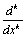µ(x) for 0 = k = J when µ(x) has (J+1) bounded derivatives. However, optimality may be defined even more stringently than the attainment of a particular convergence rate. For instance, optimality may entail minimizing mean square error or an asymptotic approximation thereto. Yet, kernel and local regression estimators ofµ(x) with minimal mean square error are not the kth order derivatives of kernel and local regression estimators of µ(x) with minimal mean square error [6,7]. While the existing literature thus provides guidance on the optimal estimation ofµ(x) by itself, or of µ(x) by itself, the existing literature does not elucidate what is optimal for the simultaneous estimation of µ(x) andµ(x) or, more generally, the simultaneous estimation of µ(x) and all of its derivatives up to order J. Here we clarify that by simultaneous we refer not merely to the explicit estimation of multiple derivatives in a single data analysis but also to the requirement that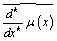and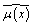honor the same functional relationship asµ(x) and µ(x), namely that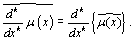Charnigo and Srinivasan  have termed this requirement "self-consistency".

There are several practical applications in which estimating a mean response function and its derivatives may help to address important scientific questions. These applications include the modeling of:

. Human height , for which the first derivative is the growth rate and the second derivative can be employed to delineate time intervals over which growth is speeding up or slowing down;

. Kidney function for a lupus nephritis patient , for which the first derivative quantifies the progress of the disease and the second derivative can be used to delineate time intervals over which the disease is progressing unstably;

. Scattering profiles of submicroscopic nanoparticles , for which the mean response function and its derivatives may be employed like "fingerprints" to identify nanoparticles of unknown size or structure given existing results for nanoparticles of known size and structure; and,

. Raman spectra of bulk materials , for which the mean response function and its derivatives may likewise be used to identify materials of unknown chemical composition. The Raman spectrum application may be particularly interesting to readers of this journal because of its potential to detect impurities as part of a quality control process in pharmaceutical production  and its potential to complement existing mammography and ultrasound technology for the noninvasive diagnosis of breast cancer via the detection of calcified lesions .

In some of these practical applications, one may reach contradictory scientific conclusions if µ(x) and its derivatives are not estimated simultaneously. For example, Charnigo and Srinivasan  illustrated the consequences of having inequalities among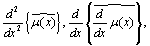and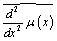in the human height application. Employing local regression, Charnigo and Srinivasan  found that the estimated first derivative for one child had a local maximum at 10.5 years, suggesting that the child's growth spurt peaked at 10.5 years. On the other hand, the estimated second derivative for that same child was nonzero at 10.5 years. The closest zero of the estimated second derivative was at 10.1 years, translating to a discrepancy of five months in pinpointing the peak of the growth spurt. While there is inherently some uncertainty about when the growth spurt peaked, acquiring two different estimates from a single data analysis is unsettling. The preceding illustration thus demonstrates that insisting upon optimal estimation of µ(x) by itself, ofµ(x)by itself, of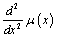by itself, and similarly for higher order derivatives may lead to incoherent scientific conclusions.

We therefore perceive the need for a new criterion by which optimality may be defined when multiple derivatives are estimated simultaneously. Such a criterion would evaluate a family of selfconsistent estimators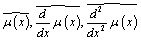and so forth rather than evaluating each estimator by itself. Ideally, this criterion would favor good estimation of several derivatives over excellent estimation of one derivative accompanied by poor estimation of the remaining derivatives. Hence, a family of estimators deemed optimal by such a criterion would not be anticipated to include, for example, athat minimized the mean square error in estimating µ(x) ; the derivatives of such awould likely be too undersmoothed to serve as good proxies for the derivatives of µ(x). Likewise, an optimal family would not be anticipated to include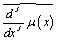that minimized the mean square error in estimatingµ(x); the antiderivatives of suchwould likely be too oversmoothed to serve as good proxies for the antiderivatives ofµ(x).

What, then, might such a criterion look like? One idea would be to consider the sum of mean square errors,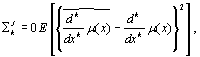with the rationale that the sum could be minimized only if each derivative were well estimated. Yet, because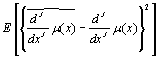might be much larger than any other summand, the sum might overemphasize the estimation ofµ(x) and thereby lead to oversmoothing in the estimation of µ(x) and its lower order derivatives. A less naive idea would be to consider a weighted sum of mean square errors,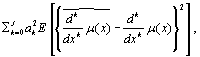or the mean square error of a weighted sum of derivative estimators,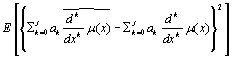. Either way, a sensible specification of a0 through aJ would be required for the criterion to serve its intended purpose. In light of Stone's  theory, one might think to let ak escalate in proportion to n(J+1-k)/(2J+3) as the sample size n increased. However, prescribing ak = ck n(J+1-k)/(2J+3) with ck not dependent on n only reduces the question of specifying a0 through aJ to the problem of choosing c0 through cJ. One might imagine that c0 = c1 = . . . = cJ = 1 would be a natural default choice and least vulnerable to criticism for appearing ad-hoc, but whether such a choice would allow the criterion to serve its intended purpose is unclear.

The considerations in the preceding paragraph are, of course, predicated on the belief that µ(x) is (J + 1) - times differentiable. A still greater challenge remains in formulating a criterion by which optimality may be defined when µ(x) is infinitely differentiable and all of its derivatives are to be estimated simultaneously. Such a criterion is motivated by the recognition that, although one may not envisage practical applications in which estimates of all derivatives are required, there may exist practical applications in which the number of derivative estimates required is not known a priori. For example, in the Raman spectrum application, a researcher may first examine an estimate of µ(x). If the estimate of µ(x) reveals the chemical composition of the material, then the researcher may stop. Otherwise, the researcher may examine an estimate of µ'(x). This process may continue, with the researcher subsequently examining estimates of µ" (x) and higher order derivatives, until the researcher either knows the chemical composition of the material or regards the higher order derivative estimates as so noisy that he/she is simply forced to make a guess about the chemical composition. Charnigo, Hall and Srinivasan  provide an example in which an estimate of µ' (x) leads to successful identification of a sample of cerium bastnasite. In general, then, the number of derivative estimates to be examined may not be known a priori. Unfortunately, since most nonparametric regression techniques make no provision for the estimation of infinitely many derivatives, there is little theory to inform the construction of a criterion by which optimality may be defined when µ (x) is infinitely differentiable and all of its derivatives are to be estimated simultaneously. We thus conclude the present editorial by calling for additional research on the simultaneous estimation of a mean response function and its derivatives when the mean response function is infinitely differentiable.

#### Acknowledgements

This material is based upon work supported by the National Science Foundation under Grant No. DMS-0706857. Any opinions, findings, and conclusions or recommendations expressed in this material are those of the authors and do not necessarily reflect the views of the National Science Foundation.

#### References

Select your language of interest to view the total content in your interested language

### Article Usage

• Total views: 12175
• [From(publication date):
March-2011 - Dec 11, 2019]
• Breakdown by view type
• HTML page views : 8368Can't read the image? click here to refresh